# Type 'void' is not assignable to type in TypeScript [Fixed]Last updated: Jan 22, 2023
5 min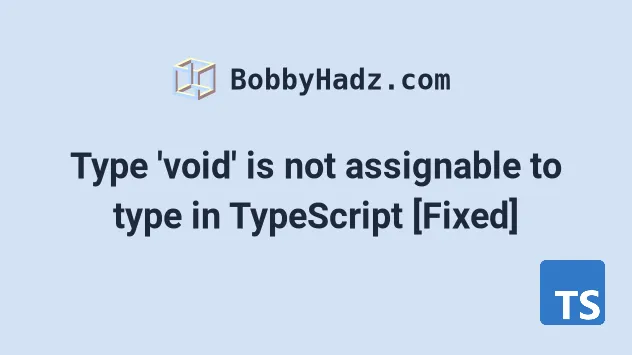If you got the error "Argument of type 'void' is not assignable to parameter of type", click on the second subheading.

## #Type 'void' is not assignable to type in TypeScript

The "Type 'void' is not assignable to type" TypeScript error occurs when we forget to return a value from a function, so the function gets an implicit return type of `void`.

To solve the error, make sure you return a value of the correct type from your functions before the assignment.

Here is an example of how the error occurs.

index.ts
```Copied!```// 👇️ const sum: (a: number, b: number) => void
const sum = (a: number, b: number) => {
const result = a + b;
// 👉️ function doesn't return anything
};

// Error: ⛔️ Type 'void' is not assignable to type 'number'.ts(2322)
const num: number = sum(50, 50);
``````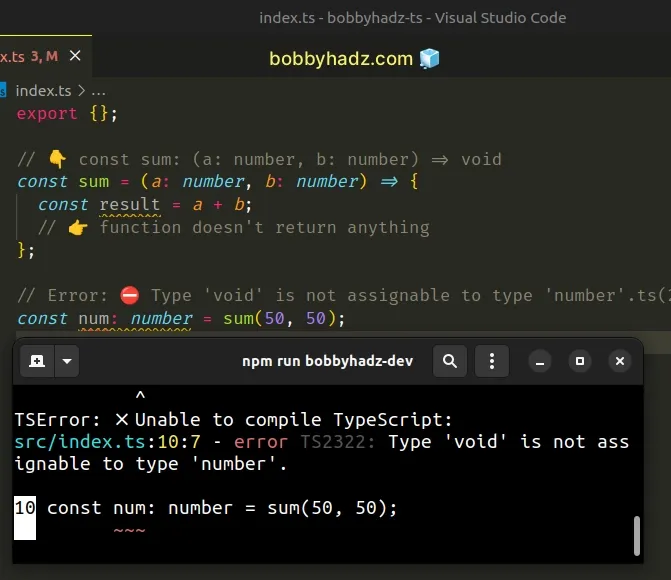We forgot to return a value from the `sum` function, so it implicitly has a return type of void.

The `void` type represents the return type of a function that doesn't return a value.

When you don't return a value from a function, you implicitly return `undefined` and the function's return type is inferred to be `void`.

The error message "Type 'void' is not assignable to type 'number'" means that we have a value that expects an assignment of type `number` and we're trying to assign a value of type `void` to it.

## #Make sure to return a value from your function

To solve the error, make sure to return a value from your function.

index.ts
```Copied!```const sum = (a: number, b: number) => {
const result = a + b;

return result; // 👈️ explicitly return
};

const num: number = sum(50, 50);

console.log(num); // 👉️ 100
``````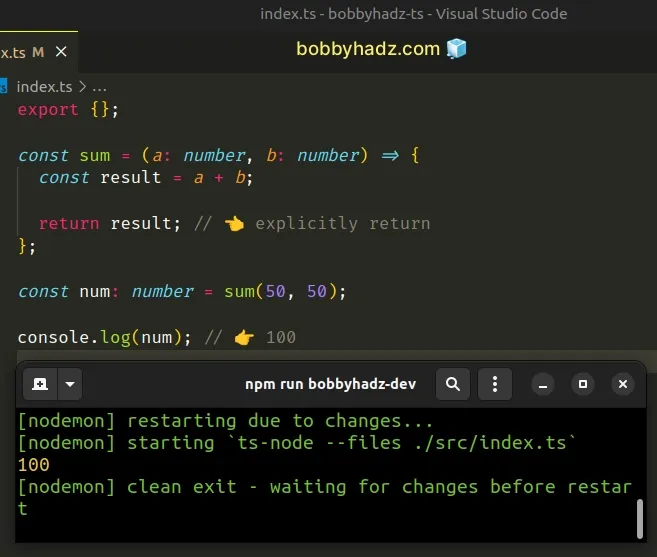Now the `sum` function has a return type of `number`.

Note that the return type of the function has to be compatible with the type of the `num` variable.

## #Explicitly set the function's return type

A helpful way to debug this is to explicitly set the function's return type.

index.ts
```Copied!```// ⛔️ Error: A function whose declared type
// is neither 'void' nor 'any' must return a value.ts(2355)
const sum = (a: number, b: number): number => {
const result = a + b;
};
``````

We explicitly set the function's return type to `number` but forgot to return a value, so TypeScript informs us that we must return a value from the function.

## #Using implicit arrow function return

If you have an implicit return in an arrow function, make sure the function returns the expected value.

index.ts
```Copied!```// 👇️ implicit return with objects
const getObj = () => ({
country: 'Chile',
});

// 👇️ implicit return with primitives
const getNum = (a: number, b: number) => a + b;
``````

## #Solve the error when working with class methods

The same error also occurs when working with class methods.

index.ts
```Copied!```class DoMath {
sum(a: number, b: number) {
const result = a + b;
}
}

const m = new DoMath();

// ⛔️ Error: Type 'void' is not
// assignable to type 'number'.ts(2322)
const num: number = m.sum(10, 15);
``````

The solution is the same - make sure that the class method returns a value of the expected type.

You might also be using callback functions that expect you to return a value of a specific type. Hover over the function and make sure to return a value of the correct type.

Sometimes you have complex functions with many conditionals and different code paths.

Note that all of the function's code paths have to return a value.

## #Argument type 'void' is not assignable to parameter of type

The error "Argument of type 'void' is not assignable to parameter of type" occurs when we forget to return from a function and pass a `void` argument to the calling function.

To solve the error, make sure you return a value of the expected type before passing it to the caller.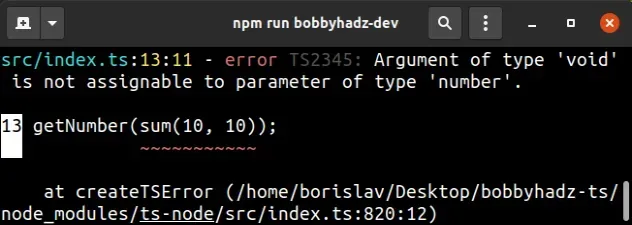Here is an example of how the error occurs.

index.ts
```Copied!```// 👇️ function sum(a: number, b: number): void
function sum(a: number, b: number) {
const result = a + b;

// 👉️ forgot to return a value
}

function getNumber(num: number) {
return num;
}

// ⛔️ Error: Argument of type 'void' is not assignable to
// parameter of type 'number'.ts(2345)
getNumber(sum(10, 10));
``````

We forgot to return a value from the `sum` function, so it implicitly has a return type of void.

The `void` type represents the return type of a function that doesn't return a value.

When you don't return a value from a function, you implicitly return `undefined`, and the function's return type is inferred to be `void`.

The error message "Argument of type 'void' is not assignable to parameter of type" means that we are passing an argument of type `void` to a function that expects a parameter of a different type.

## #Make sure to return a value from the function

To solve the error, make sure to return a value from your functions.

index.ts
```Copied!```// 👇️ function sum(a: number, b: number): number
function sum(a: number, b: number) {
const result = a + b;

return result; // 👈️ explicitly return
}

function getNumber(num: number) {
return num;
}

console.log(getNumber(sum(10, 10))); // 👉️ 20
``````Now the `sum` function has a return type of `number`.

Note that the return type of the `sum` function has to be compatible with the type of the parameter in the `getNumber` function.

A helpful way to debug this is to explicitly set the function's return type.

index.ts
```Copied!```// 👇️ explicitly set the function's return type
function sum(a: number, b: number): number {
const result = a + b;

return result; // 👈️ explicitly return
}

// 👇️ explicitly set the function's return type
function getNumber(num: number): number {
return num;
}

console.log(getNumber(sum(10, 10))); // 👉️ 20
``````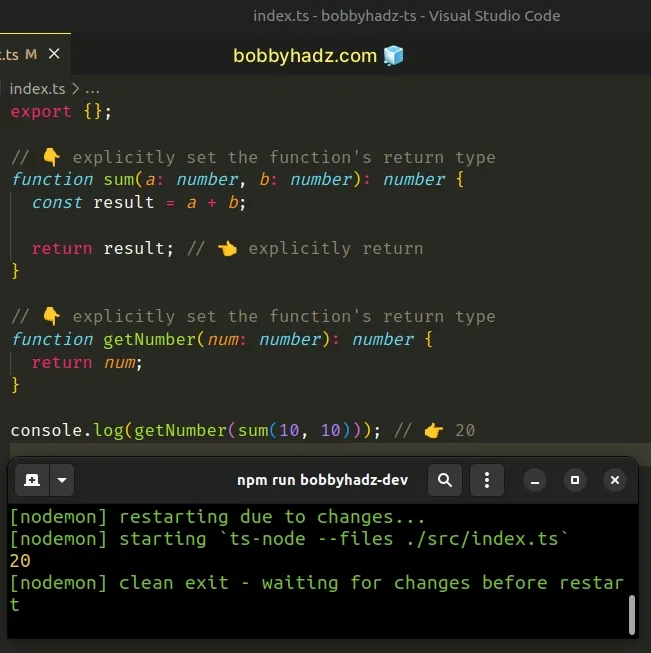We explicitly set the return type of the functions. This is useful because if the functions don't return a value of the specified type, we would get a helpful error.

## #Using arrow function implicit return

If you're using an implicit return with an arrow function, make sure the function returns the expected value.

index.ts
```Copied!```// 👇️ implicit return of object 👇️
const getPerson = () => ({
language: 'TypeScript',
});

// 👇️ implicit return 👇️
const sum = (a: number, b: number): number => a + b;

function getNumber(num: number) {
return num;
}

console.log(getNumber(sum(10, 10))); // 👉️ 20
``````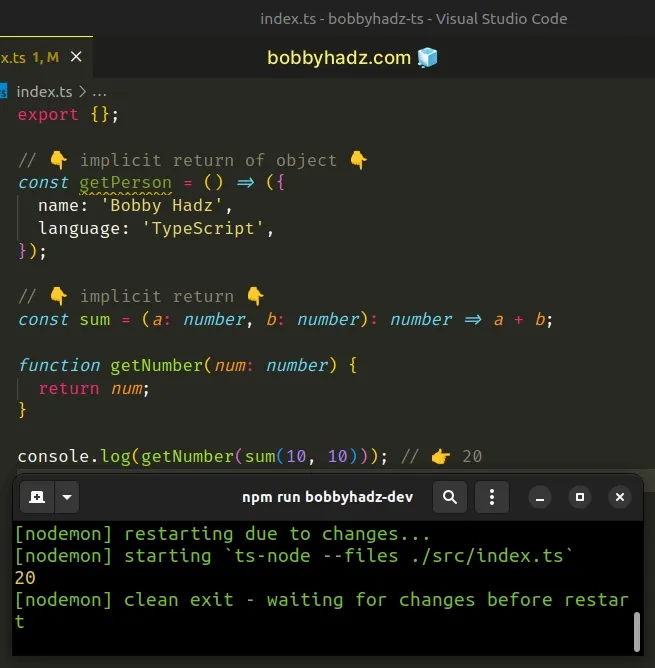You might also be using callback functions that expect you to return a value of a specific type.

Hover over the function and make sure to return a value of the correct type.

Sometimes you have complex functions with many conditionals and different code paths.

Note that all of the function's code paths have to return a value.